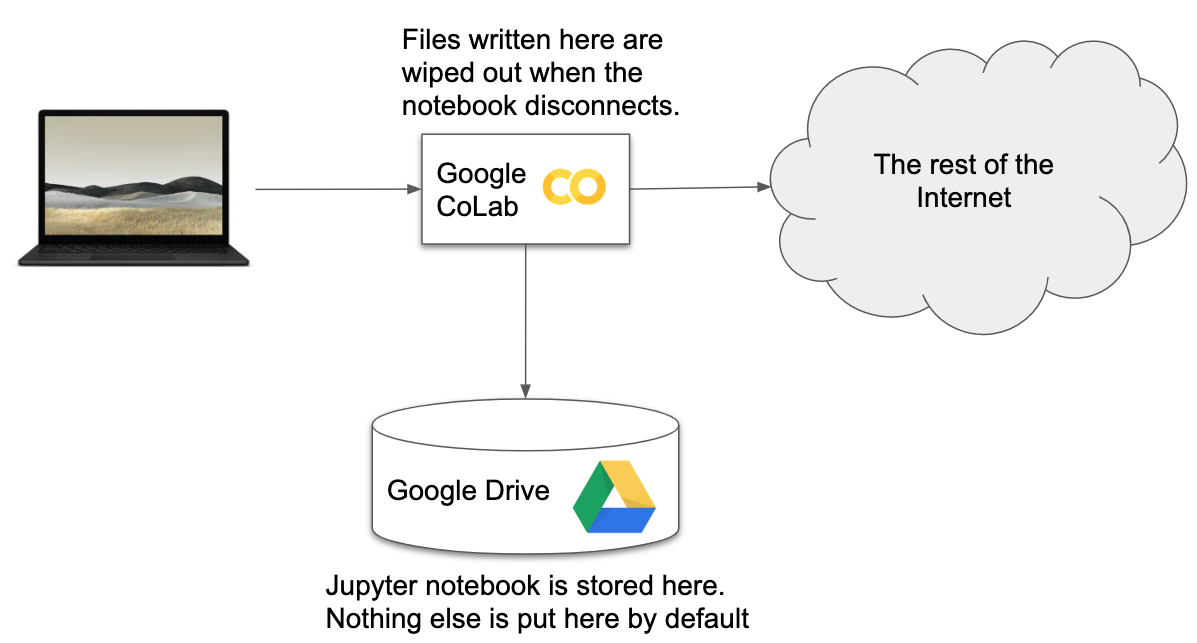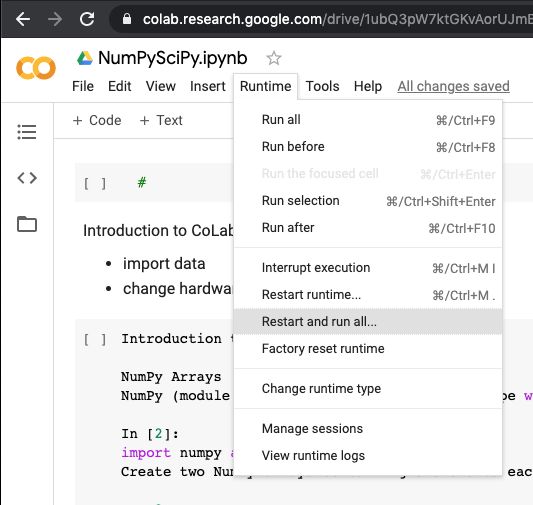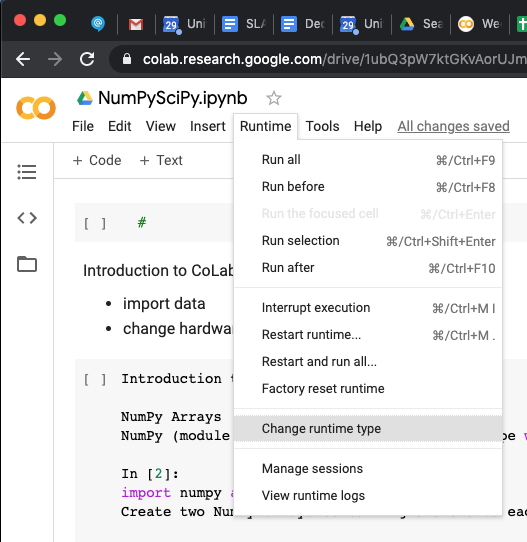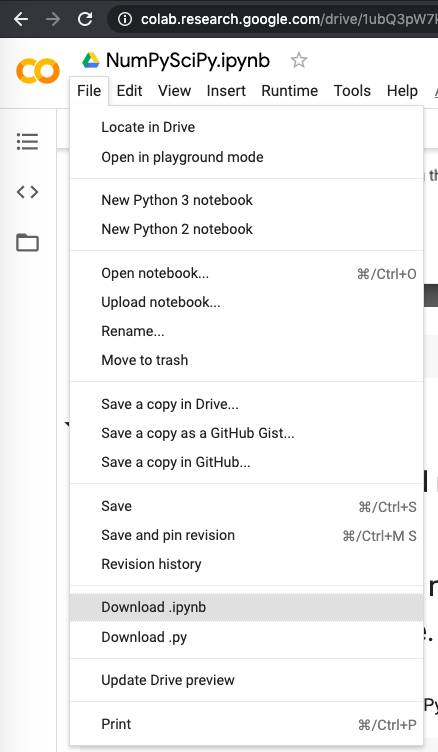# Introduction to CoLab, NumPy, and SciPy¶

### Minnesota Supercomputing Institute (MSI)¶

z.umn.edu/colab-5980

Below is a brief introduction to Google CoLab, NumPy, and SciPy. Some examples below borrow material from http://z.umn.edu/msipython and https://github.com/gmonce/scikit-learn-book

## Outline¶

• First, we're going to look at a few features in Google CoLab.
• Next, we'll look at some features of NumPy
• Finally, we'll look at a couple features of SciPyWe're going to focus on 2 evironments (CoLab and MSI). There are many others. You can run a Jupyter notebook on your own laptop or you can use other online environment like Microsoft Azure Notebooks https://notebooks.azure.com

# Moving Files¶

In :
from google.colab import drive
drive.mount('/content/gdrive')

In :
!ls /content/gdrive/
!ls "/content/gdrive/Shared drives"


Now, lets create a file and put it in our GDrive to make sure it's working.

In :
!echo woo hoo > /tmp/test.txt
!mkdir -p "/content/gdrive/My Drive/csci-5980"
!cp /tmp/test.txt "/content/gdrive/My Drive/csci-5980"


Using this connection to GDrive, we can pull data into our colab instance. Another

In :
!wget https://opendata.arcgis.com/datasets/38cfcfe07c2543ad869c8ee8c8378b5e_0.csv


now lets open it

In :
import pandas as pd


Lets try another method for getting data to our colab instance

In :
from google.colab import files

In :
uploaded = files.upload()If our instance is running on a GPU, we can take a peek at what hardware we have.

In :
!nvidia-smiIn :
!ls

In :
from google.colab import files

In :
!ls -l


Introduction to NumPy NumPy Arrays NumPy (module numpy) provides an array datatype with vectorized operations (similar to Matlab or IDL)

In :
!pip install tensorflow

In :
import numpy as np


Create two NumPy arrays containing 5 elements each. The numpy module contains a number of functions for generating common arrays:

In :
x = np.arange(5)
x

In :
y = np.ones(5)
y


Operations are vectorized, so we can do arithmetic with arrays (as long as the dimensions match!) as we would with scalar variables.

In :
x - (y+0.005) * 3


You can try to put different types of objects into an array, and NumPy will pick a data type that can hold them all.

The results might not be quite what you expect!

In :
mixed = np.array([3,3.3e-10,"string",5,5])
mixed

In :
mixed*5


More sensibly, it will choose types to avoid losing precision.

In :
z = np.array([5,6.66666666666,7,8,9],dtype=np.float32)
np.set_printoptions(precision=12)
print(z)
z.dtype


Supports the same type of list operations as ordinary Python lists:

In :
sorted(x - y * 3)


...except the data type must match! A NumPy array only holds values of a single data type.

This allows them to be packed efficiently in memory like C arrays

In :
y.dtype


Speed comparison Math with NumPy arrays is much faster and more intuitive than the equivalent native Python operations

Consider the function $y = 1.324\cdot a - 12.99\cdot b + 1$ In pure Python we would define:

In :
def py_add(a, b):
assert(len(a) == len(b))
c = *len(a)
for i in range(0,len(a)):
c[i] = 1.324 * a[i] - 12.99*b[i] + 1
return c


Using NumPy we could instead define:

In :
def np_add(a, b):
return 1.324 * a - 12.99 * b + 1


Now let's create a couple of very large arrays to work with:

In :
a = np.arange(1000000)
b = np.random.randn(1000000)

In :
len(a)

In :
b[0:20]


Use the magic function %timeit to test the performance of both approaches.

In :
%timeit py_add(a,b)

In :
%timeit np_add(a,b)


Now, we want to try out a memory profiler.

In :
!pip install memory_profiler
import memory_profiler


Oops, we don't have one installed. We can install it on-the-fly like this:

In :
import memory_profiler

In :
%memit py_add(a,b)

In :
%memit np_add(a,b)


## Spiral Data Classification¶

First, we import some modules and generate data in 3 classes

In :
%matplotlib inline
import matplotlib
from IPython.display import HTML, display, Image
import numpy as np
import matplotlib.pyplot as plt
plt.rcParams['figure.figsize'] = (12.0, 10.0) # set default size of plots
plt.rcParams['image.interpolation'] = 'nearest'
plt.rcParams['image.cmap'] = 'gray'

N = 100 # number of points per class
D = 2 # dimensionality
K = 3 # number of classes
X = np.zeros((N*K,D)) # data matrix (each row = single example)
y = np.zeros(N*K, dtype='uint8') # class labels
for j in range(K):
ix = range(N*j,N*(j+1))
t = np.linspace(j*4,(j+1)*4,N) + np.random.randn(N)*0.2 # theta
X[ix] = np.c_[r*np.sin(t), r*np.cos(t)]
y[ix] = j


### Let's visualize the data¶

In :
plt.scatter(X[:, 0], X[:, 1], c=y, s=40, cmap=plt.cm.Spectral)
plt.show()


# Train a Linear Classifier¶

## Initialize the weights with random numbers¶

In :
W = 0.01 * np.random.randn(D,K)
b = np.zeros((1,K))
step_size = 1e-0
reg = 1e-3


## Optimize the classifier using a basic gradient descent¶

In :
num_examples = X.shape
for i in range(200):

# evaluate class scores, [N x K]
scores = np.dot(X, W) + b

# compute the class probabilities
exp_scores = np.exp(scores)
probs = exp_scores / np.sum(exp_scores, axis=1, keepdims=True) # [N x K]

# compute the loss: average cross-entropy loss and regularization
correct_logprobs = -np.log(probs[range(num_examples),y])
data_loss = np.sum(correct_logprobs)/num_examples
reg_loss = 0.5*reg*np.sum(W*W)
loss = data_loss + reg_loss
if i % 10 == 0:
print("iteration %d: loss %f" % (i, loss))
dscores = probs
dscores[range(num_examples),y] -= 1
dscores /= num_examples

# backpropogate the gradient to the parameters (W,b)
dW = np.dot(X.T, dscores)
db = np.sum(dscores, axis=0, keepdims=True)

dW += reg*W # regularization gradient

# perform a parameter update
W += -step_size * dW
b += -step_size * db


## Evaluate the accuracy¶

In :
scores = np.dot(X, W) + b
predicted_class = np.argmax(scores, axis=1)
print('training accuracy: %.2f' % (np.mean(predicted_class == y)))
print(W.size)
print(b.size)


## Plot the resulting classifier¶

In :
h = 0.02
x_min, x_max = X[:, 0].min() - 1, X[:, 0].max() + 1
y_min, y_max = X[:, 1].min() - 1, X[:, 1].max() + 1
xx, yy = np.meshgrid(np.arange(x_min, x_max, h),
np.arange(y_min, y_max, h))
Z = np.dot(np.c_[xx.ravel(), yy.ravel()], W) + b
Z = np.argmax(Z, axis=1)
Z = Z.reshape(xx.shape)
fig = plt.figure()
plt.contourf(xx, yy, Z, cmap=plt.cm.Spectral, alpha=0.8)
plt.scatter(X[:, 0], X[:, 1], c=y, s=40, cmap=plt.cm.Spectral)
plt.xlim(xx.min(), xx.max())
plt.ylim(yy.min(), yy.max())


## Let's try again, and add a hidden layer¶

### We start by initializing the parameters¶

In :
h = 100 # size of hidden layer
W = 0.01 * np.random.randn(D,h)
b = np.zeros((1,h))
W2 = 0.01 * np.random.randn(h,K)
b2 = np.zeros((1,K))

step_size = 1e-0
reg = 1e-3 # regularization strength

num_examples = X.shape
for i in range(10000):

# evaluate class scores, [N x K]
hidden_layer = np.maximum(0, np.dot(X, W) + b) # note, ReLU activation
scores = np.dot(hidden_layer, W2) + b2

# compute the class probabilities
exp_scores = np.exp(scores)
probs = exp_scores / np.sum(exp_scores, axis=1, keepdims=True) # [N x K]

# compute the loss: average cross-entropy loss and regularization
correct_logprobs = -np.log(probs[range(num_examples),y])
data_loss = np.sum(correct_logprobs)/num_examples
reg_loss = 0.5*reg*np.sum(W*W) + 0.5*reg*np.sum(W2*W2)
loss = data_loss + reg_loss
if i % 1000 == 0:
print("iteration %d: loss %f" % (i, loss))

# compute the gradient on scores
dscores = probs
dscores[range(num_examples),y] -= 1
dscores /= num_examples

# backpropate the gradient to the parameters
# first backprop into parameters W2 and b2
dW2 = np.dot(hidden_layer.T, dscores)
db2 = np.sum(dscores, axis=0, keepdims=True)
# next backprop into hidden layer
dhidden = np.dot(dscores, W2.T)
# backprop the ReLU non-linearity
dhidden[hidden_layer <= 0] = 0
# finally into W,b
dW = np.dot(X.T, dhidden)
db = np.sum(dhidden, axis=0, keepdims=True)

dW2 += reg * W2
dW += reg * W

# perform a parameter update
W += -step_size * dW
b += -step_size * db
W2 += -step_size * dW2
b2 += -step_size * db2


## Evaluate training set accuracy¶

In :
hidden_layer = np.maximum(0, np.dot(X, W) + b)
scores = np.dot(hidden_layer, W2) + b2
predicted_class = np.argmax(scores, axis=1)
print('training accuracy: %.2f' % (np.mean(predicted_class == y)))
print(b.size)
print(W.size)
print(b2.size)
print(W2.size)
print(dscores.size)


### Plot the resulting classifier¶

In :
h = 0.02
x_min, x_max = X[:, 0].min() - 1, X[:, 0].max() + 1
y_min, y_max = X[:, 1].min() - 1, X[:, 1].max() + 1
xx, yy = np.meshgrid(np.arange(x_min, x_max, h),
np.arange(y_min, y_max, h))
Z = np.dot(np.maximum(0, np.dot(np.c_[xx.ravel(), yy.ravel()], W) + b), W2) + b2
Z = np.argmax(Z, axis=1)
Z = Z.reshape(xx.shape)
fig = plt.figure()
plt.contourf(xx, yy, Z, cmap=plt.cm.Spectral, alpha=0.8)
plt.scatter(X[:, 0], X[:, 1], c=y, s=40, cmap=plt.cm.Spectral)
plt.xlim(xx.min(), xx.max())
plt.ylim(yy.min(), yy.max())


Hooray, with 603 parameters, we were able to fit the data.

# Introduction to SciPy¶

### SciPy has loads of useful¶

• scipy.io.matlab – support for Matlab (.mat)
• Clustering algorithms (scipy.cluster)
• Integration and ODEs (scipy.integrate)
• Interpolation (scipy.interpolate)
• Input and output (scipy.io)
• Linear algebra (scipy.linalg)
• Muli-dimensional image processing (scipy.ndimage)
• Optimizaton and root finding (scipy.optimize)
• Signal processing (scipy.signal)
• Sparse matrices (scipy.sparse)
• Spatial algorithms and data structures (scipy.spatial)
• Special functions (scipy.special)
• Statstical functions (scipy.stats)

https://docs.scipy.org/doc/scipy/reference/tutorial/interpolate.html

1-D interpolation (interp1d) The interp1d class in scipy.interpolate is a convenient method to create a function based on fixed data points, which can be evaluated anywhere within the domain defined by the given data using linear interpolation. An instance of this class is created by passing the 1-D vectors comprising the data. The instance of this class defines a call method and can therefore by treated like a function which interpolates between known data values to obtain unknown values (it also has a docstring for help). Behavior at the boundary can be specified at instantiation time. The following example demonstrates its use, for linear and cubic spline interpolation:

In :
from scipy.interpolate import interp1d

x = np.linspace(0, 10, num=11, endpoint=True)
y = np.cos(-x**2/9.0)
f = interp1d(x, y)
f2 = interp1d(x, y, kind='cubic')

xnew = np.linspace(0, 10, num=41, endpoint=True)
import matplotlib.pyplot as plt
plt.plot(x, y, 'o', xnew, f(xnew), '-', xnew, f2(xnew), '--')
plt.legend(['data', 'linear', 'cubic'], loc='best')
plt.show()


Multivariate data interpolation (griddata) Suppose you have multidimensional data, for instance, for an underlying function f(x, y) you only know the values at points (x[i], y[i]) that do not form a regular grid.

Suppose we want to interpolate the 2-D function

In :
def func(x, y):
return x*(1-x)*np.cos(4*np.pi*x) * np.sin(4*np.pi*y**2)**2


## on a grid in [0, 1]x[0, 1]¶

In :
grid_x, grid_y = np.mgrid[0:1:100j, 0:1:200j]


### but we only know its values at 1000 data points:¶

In :
points = np.random.rand(1000, 2)
values = func(points[:,0], points[:,1])


### This can be done with the griddata function. Below, we try out all of the interpolation methods.¶

In :
from scipy.interpolate import griddata
grid_z0 = griddata(points, values, (grid_x, grid_y), method='nearest')
grid_z1 = griddata(points, values, (grid_x, grid_y), method='linear')
grid_z2 = griddata(points, values, (grid_x, grid_y), method='cubic')


### One can see that the exact result is reproduced by all of the methods to some degree.¶

In :
import matplotlib.pyplot as plt
plt.subplot(221)
plt.imshow(func(grid_x, grid_y).T, extent=(0,1,0,1), origin='lower')
plt.plot(points[:,0], points[:,1], 'k.', ms=1)
plt.title('Original')
plt.subplot(222)
plt.imshow(grid_z0.T, extent=(0,1,0,1), origin='lower')
plt.title('Nearest')
plt.subplot(223)
plt.imshow(grid_z1.T, extent=(0,1,0,1), origin='lower')
plt.title('Linear')
plt.subplot(224)
plt.imshow(grid_z2.T, extent=(0,1,0,1), origin='lower')
plt.title('Cubic')
plt.gcf().set_size_inches(10, 10)
plt.show()


# Notebooks at MSI¶

Jupyter is more than an interface for teaching. Jupyter is heavily used by researchers. It's a great interface for research. MSI has a large cluster for running large-scale simulations and data anlysis. MSI also runs JupyterHub to allow researchers to access thier data in a Jupyter environment. JupyterHub can be accessed here:

https://notebooks.msi.umn.edu

To use the notebooks at MSI, you will first need to select the group you want to work in. Most of you only have access to a single group. If you happen to be doing some research, you might have access to another group. After you select the group created for the class (csci5980) and press continue, you can click a button that reads "Start My Server". This will bring you to a dropdown menu to select the resources you need.

You can select the one labeled "Mesabi - 2 Cores, 4 GB, 8 hours". This will submit a job to the cluster and reserve the described resources for your Jupyter session.

JupyterHub at MSI is being upgraded over the next few weeks, so there will be some changes and outages to the interface. Expect email with updates!

In :